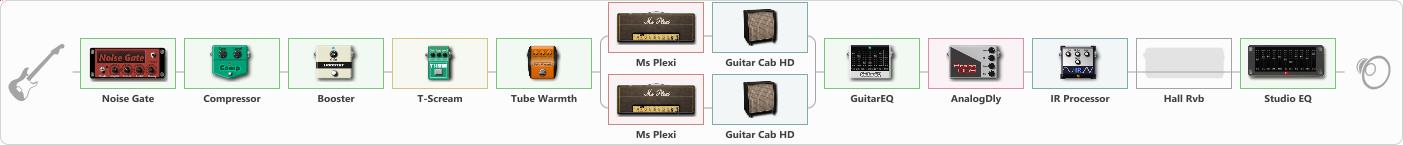# Blizzard Of Ozz UPDATED

Discussion in 'ToneLib-GFX presets' started by Tucker Stanley, Apr 9, 2023.

1. Blizzard Of Ozz UPDATED

Preset name: Blizzard Of Ozz

BEST PATCH ON GFX FOR OZZY

Effects chain:Effect: "Noise Gate" (Dynamics / Filter), active - "yes"
{
"Mode" = Auto
"Depth" = 100
"Threshold" = 96
"Attack" = 102
"Hold" = 747
"Decay" = 787
}

Effect: "Compressor" (Dynamics / Filter), active - "yes"
{
"Sense" = 89
"Level" = 100
}

Effect: "Booster" (Dynamics / Filter), active - "yes"
{
"Gain" = 43
}

Effect: "T-Scream" (Overdrive / Distortion), active - "yes"
{
"Drive" = 72
"Tone" = 72
"Level" = 84
}

Effect: "Tube Warmth" (Dynamics / Filter), active - "yes"
{
"Input" = 62
"Warmth" = 98
"Level" = 45
}

Effect: "Splitter" (Dynamics / Filter)
{
"A-Bypass" = Off
"A-Pan" = -25
"A-Level" = 55
"B-Bypass" = Off
"B-Pan" = 25
"B-Level" = 55
"Width" = 0

'A' branch:
{

Effect: "Ms Plexi" (Amp simulators), active - "yes"
{
"Gain" = 100
"Bass" = 73
"Middle" = 50
"Treble" = 55
"Presence" = 56
"Master" = 59
"Level (dB)" = 0
}

Effect: "Guitar Cab HD" (Cabinets), active - "yes"
{
"Model" = Marshall 1960A (4x12")
"Mic Model" = Dynamic SM57
"Mic Position" = Edge
"Mic Distance" = Far
"Low Cut (Hz)" = 225
"Hi Cut (kHz)" = 20.0
"Mix" = 40
"Level (dB)" = 0
}
}
'B' branch:
{

Effect: "Ms Plexi" (Amp simulators), active - "yes"
{
"Gain" = 100
"Bass" = 66
"Middle" = 39
"Treble" = 55
"Presence" = 50
"Master" = 58
"Level (dB)" = 0
}

Effect: "Guitar Cab HD" (Cabinets), active - "yes"
{
"Model" = Marshall 1960A (4x12")
"Mic Model" = Dynamic SM57
"Mic Position" = Middle
"Mic Distance" = Far
"Low Cut (Hz)" = 250
"Hi Cut (kHz)" = 6.0
"Mix" = 41
"Level (dB)" = 0
}
}
}

Effect: "GuitarEQ" (Dynamics / Filter), active - "yes"
{
"160 Hz" = 6
"400 Hz" = 1
"800 Hz" = -10
"1.6 kHz" = -4
"3.2 kHz" = 14
"6.4 kHz" = 10
"12 kHz" = 15
"Level (dB)" = 10
}

Effect: "AnalogDly" (Delay), active - "yes"
{
"Time" = 1
"Feedback" = 57
"Tone" = 100
"Mix" = 49
}

Effect: "IR Processor" (Cabinets), active - "yes"
{
"IR" = RANDY
"Low Cut (Hz)" = 250
"Hi Cut (kHz)" = 2.0
"Mix" = 100
"Level (dB)" = 0
}

Effect: "Hall Rvb" (Reverberation), active - "no"
{
"Time" = 1.3
"PreDelay" = 0
"LoDamp" = 7
"HiDamp" = 7
"Mix" = 29
}

Effect: "Studio EQ" (Dynamics / Filter), active - "yes"
{
"31 Hz" = 15
"62 Hz" = -15
"125 Hz" = -15
"250 Hz" = -11
"500 Hz" = -4
"1 kHz" = 4
"2 kHz" = 2
"4 kHz" = -9
"8 kHz" = 7
"16 kHz" = -3
"Level (dB)" = 0
}

Note: You will need to download and install the ToneLib-GFX software to use the preset.

#### Attached Files:

• ###### Blizzard_Of_Ozz_UPDATED.tlgfx
File size:
22.3 KB
Views:
3,356
dragoda, Darius Netzer and Bronepooz like this.